# ICSE Worksheet for Chapter - 11 Lines And Angles Class 8

## Find ICSE Worksheet for chapter-11 Lines And Angles class 8

1. CLASS-8
2. BOARD: ICSE
3. Mathematic Worksheet - 11
4. TOPIC: Lines And Angles
5. For otherICSE Worksheet for class 8 Mathematic check out main page of Physics Wallah.

#### SUMMARY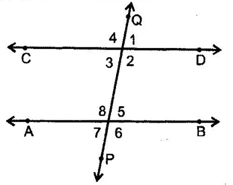• Corresponding angles: ∠1 &∠5, ∠4 &∠8, ∠2 &∠6, ∠3 &∠7 are pairs of corresponding angles.
• Alternate interior angles: ∠3 &∠5, ∠2 &∠8 are the pairs of alternate interior angles.
• Consecutive interior angles: ∠2 &∠5, ∠3 &∠8, are pairs of consecutive interior angles.
• Vertically opposite angles: in figure, ∠1 = ∠3, ∠2 = ∠4, ∠5 = ∠7 &∠6 = ∠8.

#### SECTION – I(OBJECTIVE)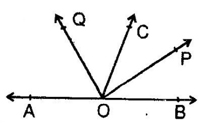• In the given fig if l II m, n II p and ∠1 = 85° then ∠2 is
1. 95°
2. 110°
3. 135°
4. 85°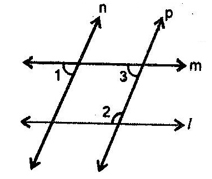• An larger angle is 14° more than its complementary angle then angle is:
1. 38°
2. 52°
3. 50°
4. none of these
• X lies in interior of ∠BAC. If ∠BAC = 70° and ∠BAX = 42° then ∠XAC = ?
1. 28°
2. 29°
3. 27°
4. 30°
• If the supplement of an angle is three times its complement, then angle is:
1. 40°
2. 35°
3. 50°
4. 45°
• Two angles whose measures are a & b are such that 2a – 3b = 60°, if they form a linear pair then find4a/5b.
1. 0
2. 8/5
3. 1/2
4. 2/3
• The supplement of an angle is one third of itself. Then the angle is:
1. 135°
2. 45°
3. 140°
4. None
• In given figure, if ∠BOC = 7x + 20° and ∠COA = 3x, then the value of x for which AOB becomes a straight line.
1. 15°
2. 12°
3. 16°
4. None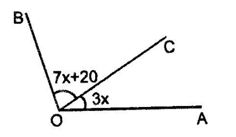• In given figure if AB II DF, AD II FG ∠BAC = 65°, ∠FGH is:
1. 120°
2. 125°
3. 115°
4. 140°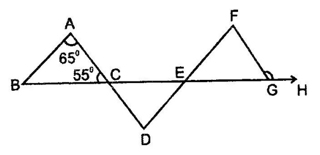• In given figure, AB II ED and ∠ABC = 30°, ∠EDC = 70° then x is:
1. 240°
2. 125°
3. 260°
4. None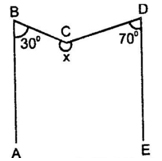• In the given figure, if EC II AB, ∠ECD = 70° and ∠BDO = 20°, then ∠OBD is-
1. 20°
2. 50°
3. 60°
4. 70°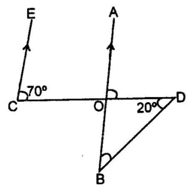• Given AB II CD, x° =
1. 20°
2. 70°
3. 110°
4. 35°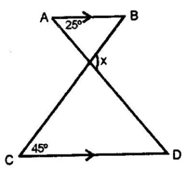• As shown in the figure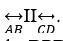. If point P is between these two lines such that m∠ABP = 50° and m∠CDP = 70° then find ∠BPD.
1. 120°
2. 70°
3. 110°
4. 50°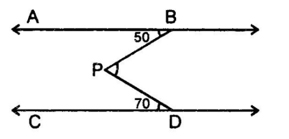• The measure of that angle which is four times its supplement is:
1. 36°
2. 144°
3. 155°
4. 180°

1. A
2. B
3. A
4. A
5. B
6. A
7. D
8. B
9. A
10. C
11. B
12. C
13. B
14. B
15. A
16. B